# 【数学】三边相等的四边形与60度角

$\Huge\textsf{三边相等的四边形与60}\degree\textsf{角}$





$\Large\texttt{例<1>}$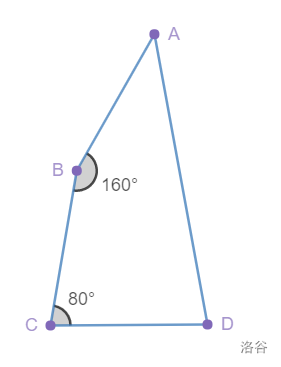$CD$为边向上作正三角形$CED$，连接$AE$，则 $AB = BC = CD = CE = ED$

$\angle BCE = \angle BCD - \angle ECD = 80\degree - 60\degree = 20\degree = 180\degree - \angle B ,\;\therefore AB \parallel CE$

$\because AB = BC = CE,\;\therefore \;$四边形$ABCE$为菱形，$\therefore AE = …… = ED .$

$\angle AED = 360\degree - \angle AEC - \angle CED = 360\degree - 160\degree - 60\degree = 140\degree$

$\therefore \angle EAD = \angle EDA = (180\degree - 140\degree)/2 = 20\degree$

$\therefore \angle BAD = \angle BAE + \angle EAD = 20\degree + 20\degree = 40\degree .$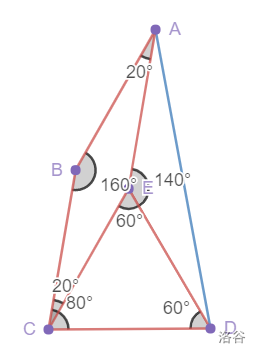$\Large\texttt{例<2>}$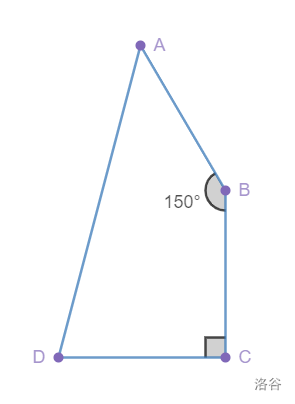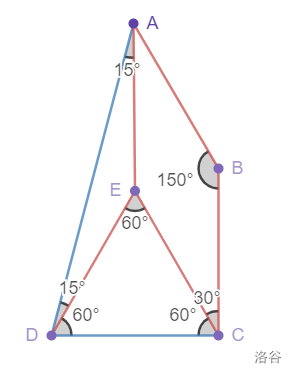$\therefore \angle AEC = \angle B = 150\degree ,\;\angle EAB = \angle ECB = 30\degree .$

$\because \angle AEC = 150\degree , \;\angle DEC = 60\degree ,\;\therefore \angle AED = 150\degree ,$

$\because EA = ED,\;\therefore \angle EAD = \angle EDA = 15\degree .$

$\therefore \angle A = \angle DAE + \angle BAE = 15\degree + 30\degree = 45\degree .$



$\Large\texttt{例<3>}$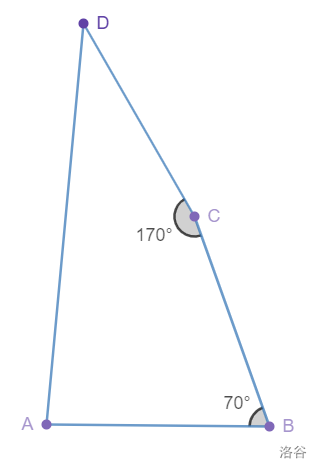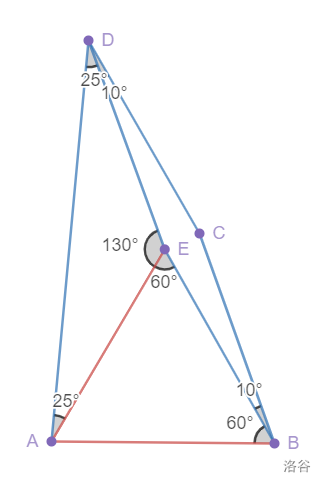### 在凸四边形$ABCD$中，$AB=BC=CD,\angle B +\angle C = 240\degree ,$则剩下两个角较小的一个为$\frac{1}{2} \angle B.$



$\large\textsf{小拓展！}$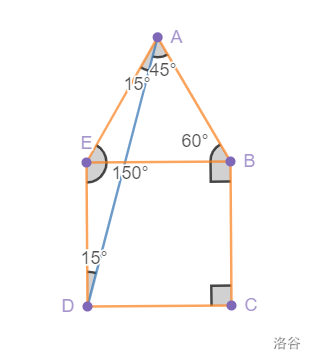$\angle ABE = \angle ABC - \angle EBC = 60\degree ,\;$$AB = BC = BE ,\;\therefore \triangle ABE$为正三角形。

$\therefore \angle AED = 60\degree + 90\degree = 150\degree ,\;$又因为$AE = AD ,\;\therefore \angle EAD = \angle EDA = 15\degree ,$

$\therefore \angle DAB = \angle EAB - \angle EAD = 60\degree - 15\degree = 45\degree .$





$\Large\texttt{例<4>}$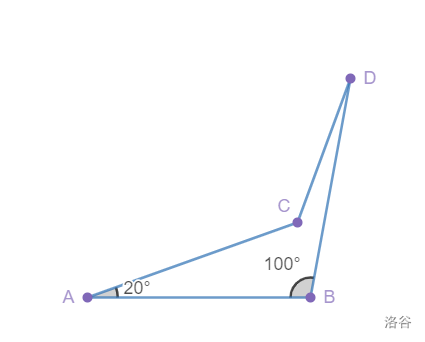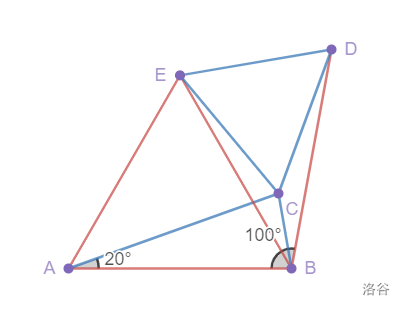$\therefore \angle ACB = \angle ABC = 80\degree ,\;\angle ACE = \angle AEC = 70\degree ,\angle ECB = \angle ACE + \angle ACB = 150\degree .$

$\angle DBE = \angle ABD - \angle ABE = 40\degree ,\;\angle DBC = \angle ABD - \angle ABC = 20 \degree ,\;\therefore \angle DBC = \angle EBC = 20\degree$

$\because EB = DB ,\;CB$为公共边，$\therefore \triangle CBE \cong \triangle CBD \;(SAS)$

$\therefore \angle DCB = \angle ECB = 150\degree ,\;\angle ECD = 360\degree - \angle DCB - \angle ECB =60\degree .$

$\because EC = DC ,\;\therefore \triangle ECD$为等边三角形$,\;\therefore \angle EDC = 60\degree .$

$\therefore \angle CDB = \angle EDB - \angle EDC = 10\degree .$



$\Large\texttt{例<5>}$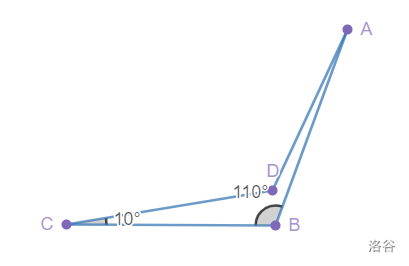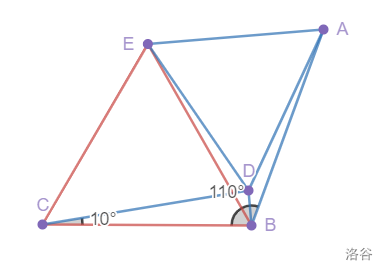$\triangle ECD$为等边三角形$,\;\angle DAB = \angle EAB - \angle EAD = 5\degree .$

### 在凹四边形$ABCD$中，$AB=BC=CD,\angle B +\angle C = 120\degree ,$则剩下的锐角为$\frac{1}{2} \angle C.$$\Large\texttt{例<5>}$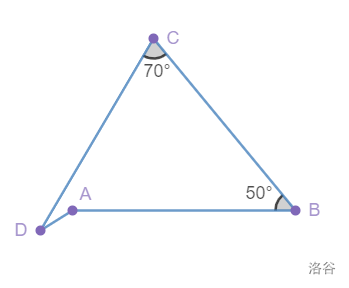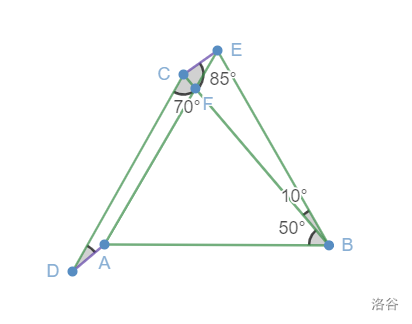$AE = BE = AB = BC = CD ,\;\angle EBC = \angle EBA - \angle CBA = 10\degree$

$\therefore \angle AFB = \angle FEB + \angle FBE = 60\degree + 10\degree = 70\degree = \angle DCB ,\;\therefore CD \parallel EA ,\;$

$\angle BCE = \angle BEC = 85\degree ,\;\therefore \angle DCE = 155\degree .$

$\because CD = EA ,\;\therefore CDAE\;$ 是平行四边形！

$\therefore \angle D +\angle ACE = 180\degree ,\;\angle D = 25\degree !$



$\Large\texttt{例<6>}$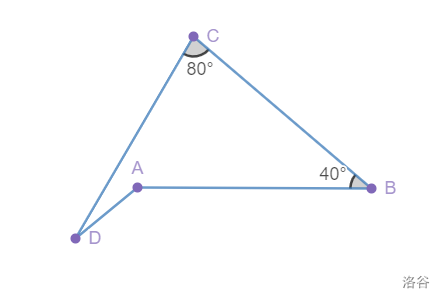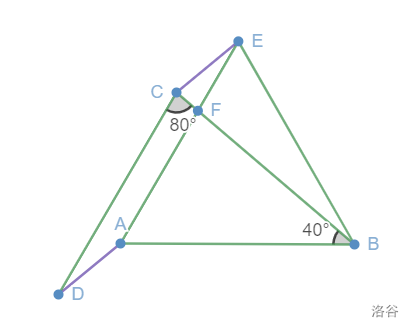### 在凹四边形$ABCD$中，$AB=BC=CD,\angle B +\angle C = 120\degree ,$则剩下的锐角为$\frac{1}{2} \angle B.$



$\Large\texttt{例<7>}$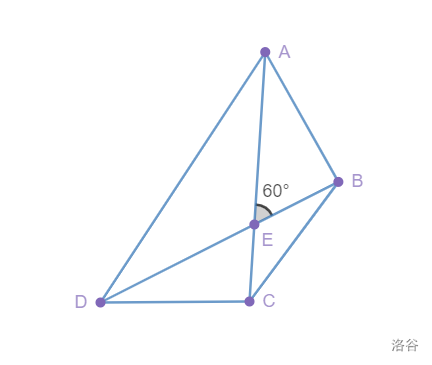$\large\texttt{法<1>}$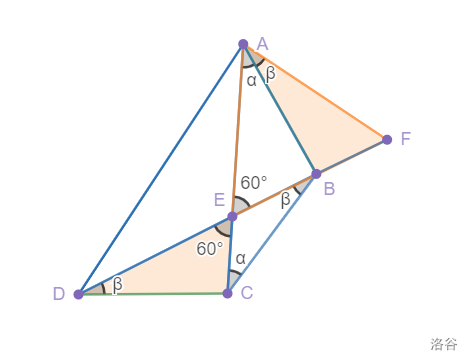$\angle EAB = \angle ECB = \alpha ,\;\angle BDC = \angle DBC = \beta.$

$\triangle BEC$ 中，$\angle ECB + \angle EBC = \angle AEB$，即$\alpha + \beta = \angle AEB = 60\degree ;$

$\angle EAB + \angle FAB = \angle EAF$，即$\alpha + \angle BAF = 60\degree ,\;\therefore \angle BAF = \beta .$

$\angle FAB = \angle EDC =\beta ,\;BA = CD ,\; \angle F = \angle DEC = 60\degree ,\; \therefore \triangle FAB \cong \triangle EDC ,$

$\therefore EA = AE = ED .$ 得证。



$\large\texttt{法<2>}$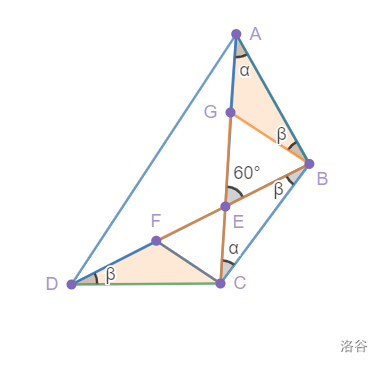$\therefore EA = AG + GE = AG + GB = FC + DF = FE + DF = DE .$得证。



$\large\texttt{小拓展！}$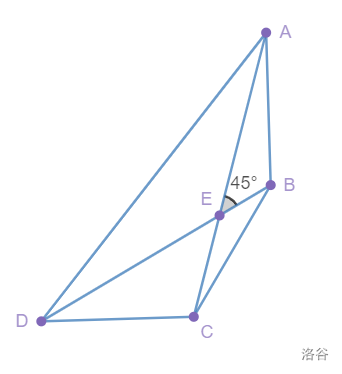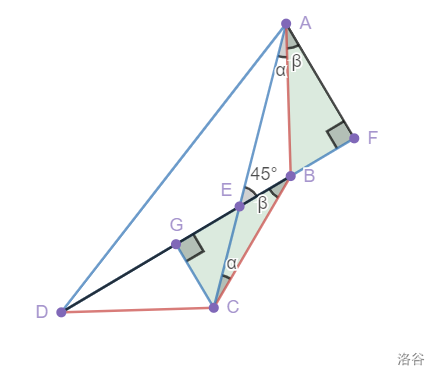$\angle EAB = \angle ECB = \alpha ,\;\angle BDC = \angle DBC = \beta.$

$\triangle BEC$ 中，$\angle ECB + \angle EBC = \angle AEB$，即$\alpha + \beta = \angle AEB = 45\degree ;$

$\angle EAB + \angle FAB = \angle EAF$，即$\alpha + \angle BAF = 45\degree ,\;\therefore \angle BAF = \beta .$

$\angle FAB = \angle GBC =\beta ,\;BA = CB ,\; \angle F = \angle CGB = 90\degree ,\; \therefore \triangle FAB \cong \triangle GBC ,\;\therefore AF = BG.$

$EA = \sqrt{2} AF,\;BD = 2BF ,\;\therefore BD = \sqrt{2}AE.$ 得证。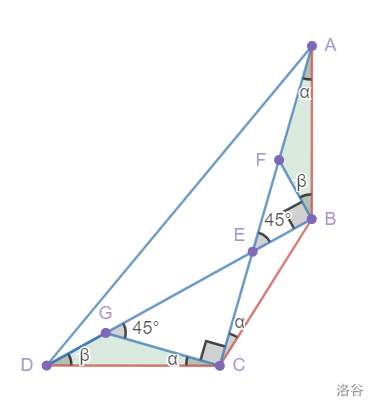$\triangle DGC \cong \triangle BEC ,\;\therefore BE = DG,$

$\therefore AE = AF + FE = GC + \sqrt{2}BE = \frac{\sqrt{2}}{2}(GE + 2BE) = \frac{\sqrt{2}}{2} BD .$



$\huge\textsf{总结}$

$\small\texttt{Summary!}$





1.画图软件：Desmos

2.参考资料：暂时没在网络上找到相应资料

3.转载请注明出处 $\tiny\textbf{（虽然应该不会有人来抄我这个小菜鸡的文章XD）}$

©️2019 CSDN 皮肤主题: 深蓝海洋 设计师: CSDN官方博客